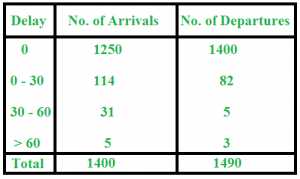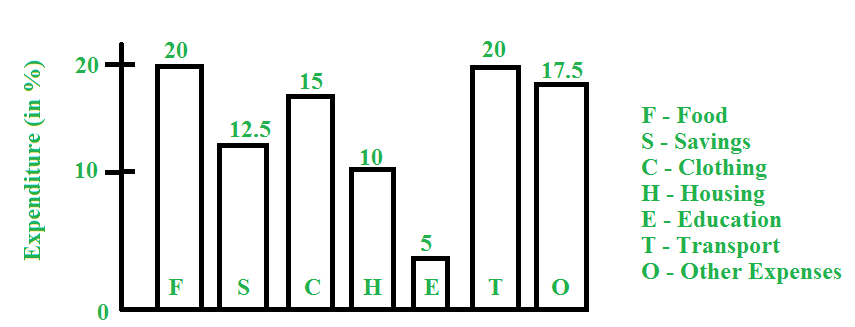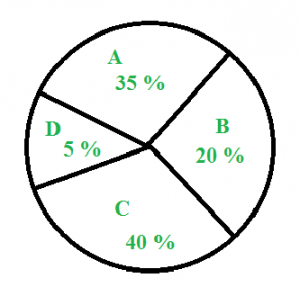# Data Interpretation

In data interpretation, we are given data in the form of visuals, like table, graph, chart, etc. and a set of questions that follow. Mainly, they use percentage , ratio or proportion.
Here, we discuss some commonly asked questions.

### Tables

Question : The following table contains the data of train arrival and train departure from a station. Study the table and answer the questions that follow :a) Find the number of trains that arrived late
b) Find the number of trains that departed late
c) Find the percentage of late arriving trains
Solution :
a) Number of trains arriving late = 114 + 31 + 5 = 150
b) Number of trains departing late = 82 + 5 + 3 = 90
c) Percentage of late arriving trains = (150 / 1400) x 100 = 10.71 %

### Bar Graph

Question : Study the following bar graph and answer the questions that follow :
Total monthly income = Rs. 50,000a) What amount is spent on food ?
b) How much more money is spent on clothing and housing together than on transportation?
c) What percent of amount on food is spent as amount on education?
Solution :
a) Food = 20 % of expenditure
=> Amount spent on food = 20% of 50,000 = Rs. 10,000
b) Money spent on clothing and housing together = 25 % of Rs. 50,000 = Rs. 12,500
Money spent on transportation = 20 % of Rs. 50,000 = Rs. 10,000
Therefore, more money spent on clothing and housing together than on transportation = Rs. 12,500-10,000 = Rs. 2,500
c) Expenditure on food = 20 %
Expenditure on education = 5 %
Therefore, percent of amount on food spent as amount on education = (5 / 20) x 100 = 25 %

### Pie Chart

Question : Study the pie chart below and answer the questions that follow :The above pie chart shows the sales of four different types of articles in a shop.
a) What is the central angle of type A ?
b) If the total sale is 1200, what is the sale of B ?
c) What is the difference between the central angle of C and D ?
Solution :
a) Central angle of A = Percentage of A x 360 degrees = (35 / 100) x 360 = 126 degrees
b) Sales of B = 20 % of 1200 = 240
c) Difference between the central angle of C and D = 40 % of 360 – 5 % of 360 = 35 % of 360 = 126 degrees

Please write comments if you have any doubts related to the topic discussed above, or if you are facing difficulty in any question or if you would like to discuss a question other than those mentioned above.